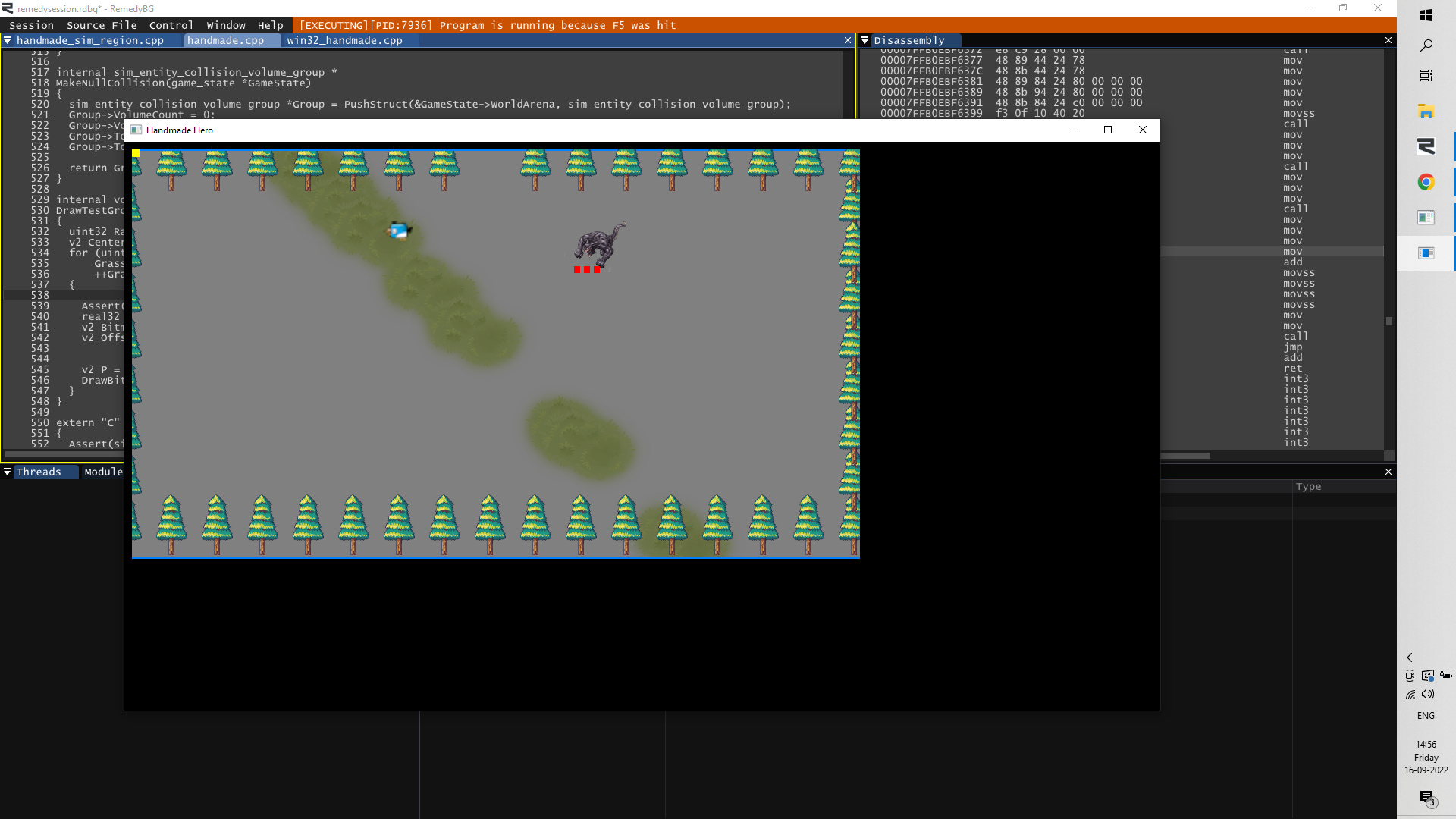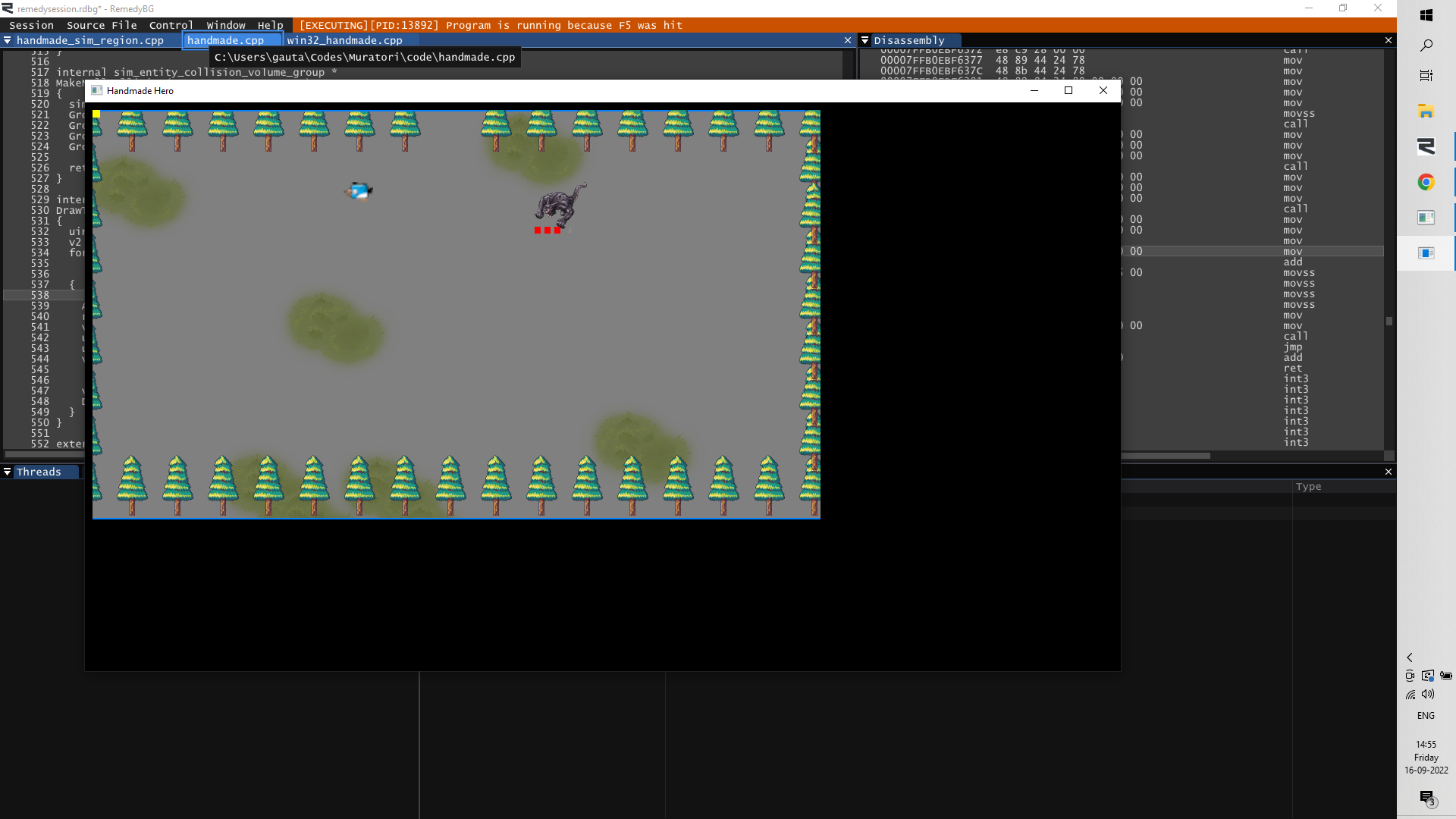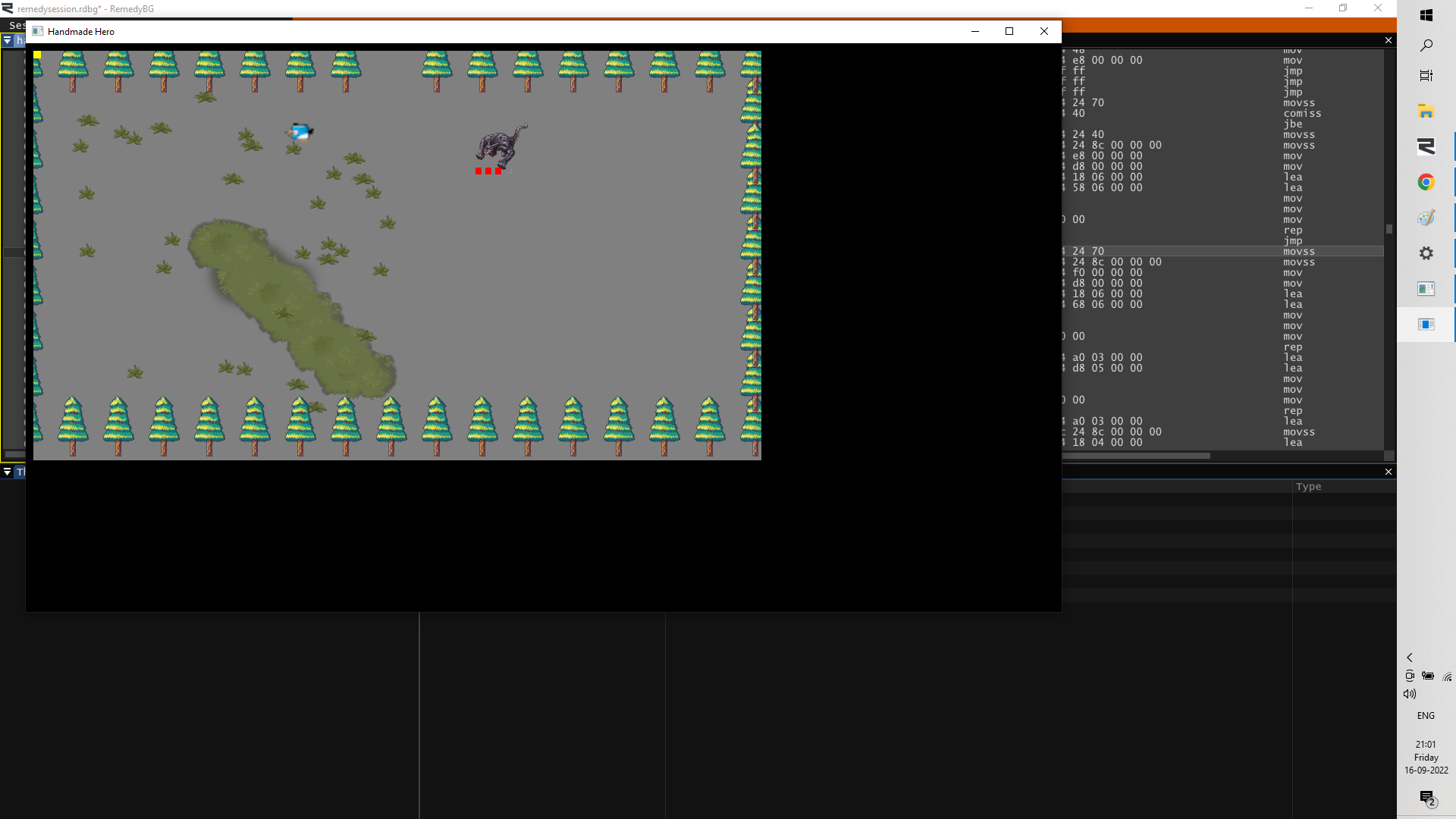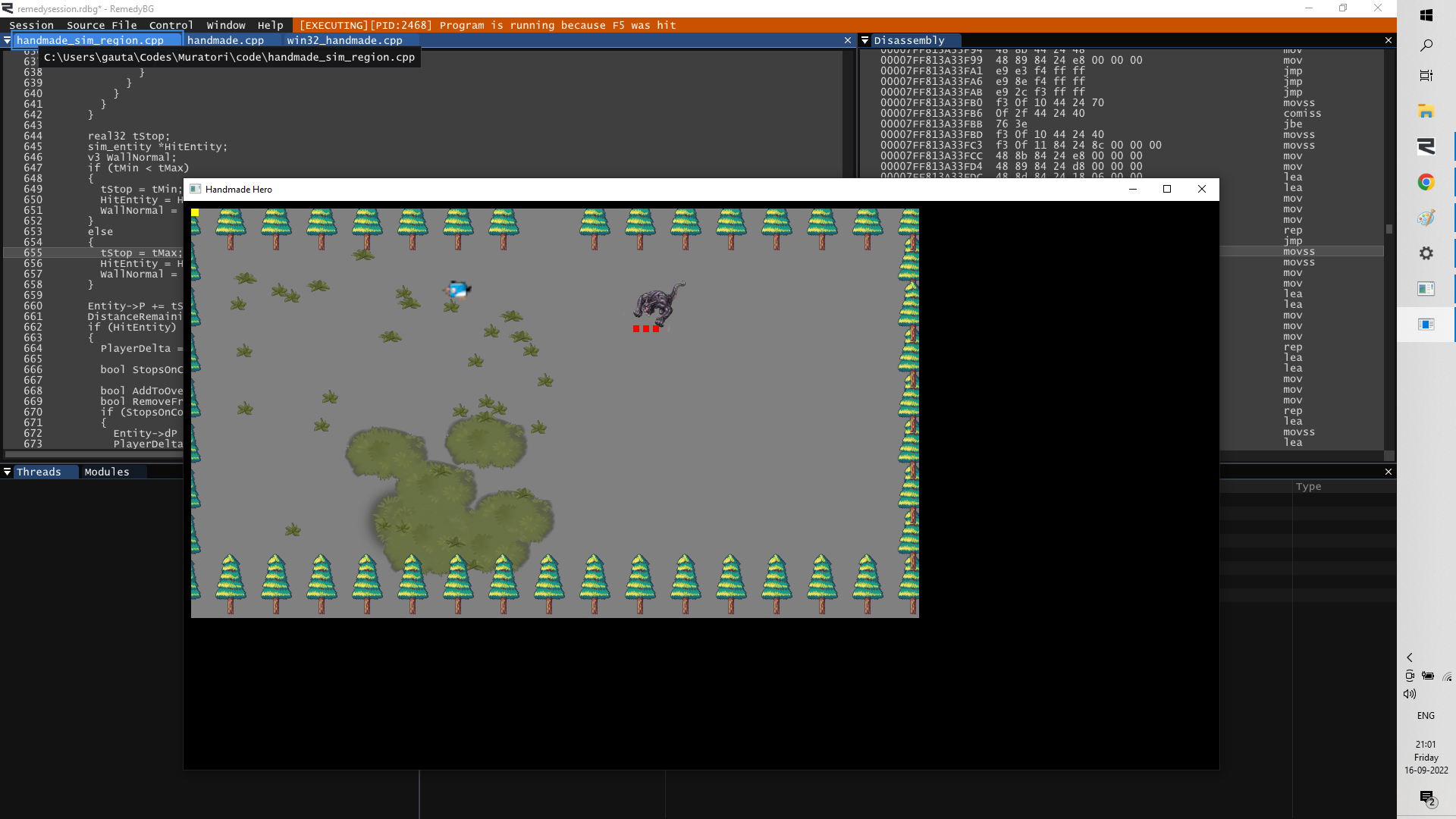Gaurav Gautam
93 posts
Day81: The random generation of X and Y coordinates is a little bit wrong.
Edited by Gaurav Gautam on

Hi Simon,

On Day 81 the ground bitmaps were loaded and their X and Y coordinates were randomly generated with random numbers. The expression used on the stream was this

    v2 Offset = V2(2.0f * ((real32)RandomNumberTable[RandomNumberIndex++] / (real32)MaxRandomNumber) - 1.0f,
2.0f * ((real32)RandomNumberTable[RandomNumberIndex++] / (real32)MaxRandomNumber) - 1.0f);


In this expression the division is basically assuming that the minimum number is 0. In that case the numerator/denominator will give a number between 0 and 1 randomly distributed. However, thats not the case as we saw on the stream. Although in the stream's case the lower number is close to 0, making this almost correct. And when I use this code (with my random number table) I get thisI am not sure why this is on a straight line though. The random numbers I generated came out with a higher maximum than Casey Muratori's. And Im guessing its because of that, but I can't explain why that would happen.

My min and max are these

#define MaxRandomNumber 0x5f5b1ea
#define MinRandomNumber 0x5287


When I modify the expression to subtract the minimum number from the numerator and denominator the grass is laid out properly. Here is the expression I used:

    uint32 XIndex = RandomNumberIndex++;
uint32 YIndex = RandomNumberIndex++;
v2 Offset = V2(2.0f * ((real32)(RandomNumberTable[XIndex] - MinRandomNumber) / (real32)(MaxRandomNumber - MinRandomNumber)) - 1.0f,
2.0f * ((real32)(RandomNumberTable[YIndex] - MinRandomNumber) / (real32)(MaxRandomNumber - MinRandomNumber)) - 1.0f);


And the result from this isSo, can you tell me why would that make a straight line?

P.S. Actually I found later in the qna part of the stream that he did point out the subtraction as in my expression above.

507 posts
Day81: The random generation of X and Y coordinates is a little bit wrong.
Edited by ratchetfreak on

My gut feeling is that you are hitting the undefined behavior related to when a post increment gets committed to a variable vs. when it gets loaded for the value when 2 of them happen in the same statement.

This would lead to the same index being used twice

real32 realx = (real32)RandomNumberTable[RandomNumberIndex++]/(real32)MaxRandomNumber;
real32 realy = (real32)RandomNumberTable[RandomNumberIndex++]/(real32)MaxRandomNumber;
v2 Offset = V2(2.0f * realx  - 1.0f,
2.0f * realy  - 1.0f);


should fix that particular bug.

or get the index explicitly like you did in your second statement.

Gaurav Gautam
93 posts
Day81: The random generation of X and Y coordinates is a little bit wrong.
Edited by Gaurav Gautam onWith the indices calculated separatelyThankyou for explaining. I did set a breakpoint there and try to figure this one out but I didn't even suspect that the value of RandomNumberIndex++ itself could be the culprit. Nor would I have had any clue about how to look at its two values in the same expression. And separating it out from the expression would have made the bug disappear 😅.